# Lagrange multipliers and their physical meaning

Source: Test

Lagrange multiplier-y learning time

After the immensely-practical torque calculation series, let’s do something completely different: intricacies of numerical analysis. Yay!

Are you familiar with Lagrange multipliers? Besides their obvious use in optimization, they are also quite handy in finite element analysis. Like in optimization, their purpose in FEA is enforcing boundary conditions and other constraints. However, to get correct results, it’s kinda important that you don’t just blindly throw them into the mix.

### Sliding interface

I first came across them in an electric motor simulation. They were used to enforce continuity between separate stator and rotor meshes, across a so-called sliding interface. The continuity was defined based on something called the polynomial interpolation method, but that’s not important right now. What IS important is that the continuity condition looked like something as simple as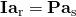.

In other words, the potential on rotor-side of airgap was set to equal to stator-side.

In addition, the basic FEA equation of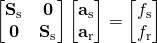should of course be satisfied.

Now, I was in a hurry to get things done, so I dug only a little deeper. I found out that I could simply introduce new variables (let’s call them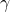), and solve the following system instead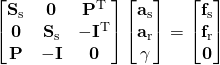.

That worked, so I mentally filed it under ‘Matrix Magic’ and never thought about it until much, much later.

By the way, that’s exactly the very same sliding interface condition that I mentioned in the numerical torque computation post.

##### 3D analysis

That time came when I was considering some 3D analysis, using the vector potential (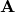) formulation. To get a unique solution, the following gauge condition should be satisfied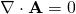.

I happened to know that an easy way to achieve this would be with Lagrange multipliers, again. So, it’d make sense that the final matrix equation would look like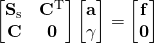.

In other words, simply add the constraint equationin matrix form, and then some matrix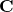to couple them together, right?

Right?

Except it wouldn’t.

Why?

Now that’s the question.

### Physical meaning of lil-L

And to understand that, we have dig deeper into the physical meaning of, well, everything. So not simply Matrix Magic anymore – yuck!

Let’s begin with the gauge condition.

It’s there because the vector potential isn’t unique in 3D. We can add any gradient of a scalar potential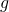to it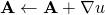and still get the same field solution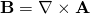.

It other words, A can be thought to contain an unknown gradient field.

Now, the correct approach is to add another include this gradient field as an unknown – to solve bothand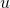and then require that their sum satisfies the gauge condition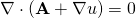.

By looking at the two equations, it’s quite easy to guess that the resulting matrix equation will look like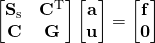.

So, we have an extra block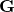in the lower right corner.

Furthermore, now we understand why everything is as it is. The Lagrance multipliers (now called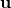!) aren’t an artificial constraint anymore. Instead, they directly define a potential, in effect cancelling out the gradient part hidden inside A.

##### Original example

So, how does this relate to out original example?

Well, it turns out the multipliershave a clear physical meaning there as well. Specifically, the add an additional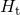– tangential component of the magnetic field the airgap boundaries of the stator and rotor. Hence the appearance of the matrices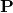and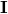on the first two rows of the linear system.

Moreover, the tangential field is defined to be such that the solution is then continuous over the airgap interface. Thus the third, extra row.

Wondering why get the relatively weird tangential field? That’s simply a peculiarity of the vector potential. For many other problems, we’d see a normal component – such as a heat flux for thermal problems, or boundary force for mechanics.

##### Another case

Analysing an electric machine with circuit connections? Whether you know it or not (still using black-box commercial software?), some voltages are being solved along with the field quantities. And know what, these voltages are are in fact nothing more than Lagrance multipliers for the field problem.

### Conclusion

• Lagrance multipliers are a handy way of adding constraints to an FEA problem.
• Despite their misleading name, the multipliers do have a physical meaning.
• Blindly adding constraints (ignoring the point above) can lead to an incorrect solution.

The post Lagrange multipliers and their physical meaning appeared first on Antti Lehikoinen.